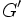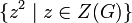# Group whose derived subgroup is contained in the square of its center

This article defines a group property: a property that can be evaluated to true/false for any given group, invariant under isomorphism
View a complete list of group properties
VIEW RELATED: Group property implications | Group property non-implications |Group metaproperty satisfactions | Group metaproperty dissatisfactions | Group property satisfactions | Group property dissatisfactions

## Definition

A group$G$ is termed group whose derived subgroup is contained in the square of its center if it satisfies the following equivalent conditions:

1. For every$x,y \in G$, there exists$z$ in the center$Z(G)$ such that$z^2 = [x,y]$, where$[x,y]$ denotes the commutator of$x$ and$y$.
2. The derived subgroup$G'$ is contained in the subgroup$\{z^2 \mid z \in Z(G) \}$ where$Z(G)$ is the center of$G$.

## Relation with other properties

### Weaker properties

Property Meaning Proof of implication Proof of strictness (reverse implication failure) Intermediate notions